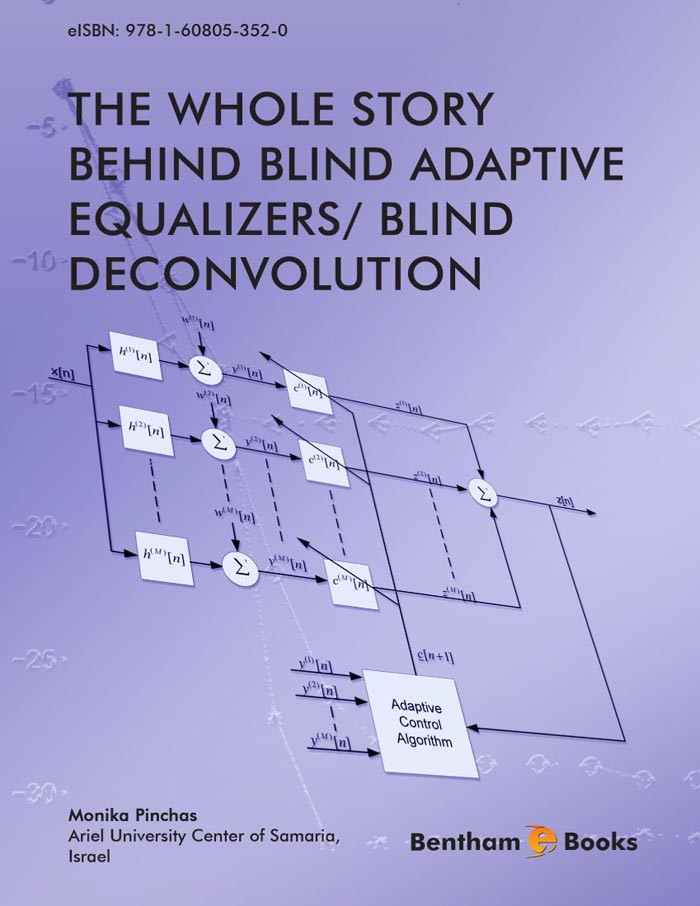### The Convergence Time of A Blind Adaptive Equalizer

#### Abstract

Closed-form approximated expressions were recently proposed for the convergence time (or number of iterations required for convergence) and for the Intersymbol Interference (ISI) as a function of time valid during the stages of the iterative deconvolution process. The new derivations are valid for the noiseless, real valued and two independent quadrature carrier case and for type of blind equalizers where the error that is fed into the adaptive mechanism which updates the equalizer&#039;s taps can be expressed as a polynomial function of order three of the equalized output like in Godard&#039;s algorithm. The derivations are based on the knowledge of the initial ISI and channel power (which is measurable) and do not need the knowledge of the channel coe cients. However, they are not based on strong mathematical foundations and were tested via simulation with Godard&#039;s algorithm for two dierent channels only. Thus, it could be argued that they hold only for a particular equalization method and for special cases. In this chapter, we present by simulation the usefulness of the recently derived expressions for more channel types and step-size parameters as well as for another type of equalizer. Thus, the question if the obtained expressions hold also for other types of equalization methods and other channels is answered. &lt;/P&gt; &lt;P&gt; Based on the recently proposed expression for the ISI as a function of time, we derive in this chapter a closed-form approximated expression for the mean square error (MSE) as a function of time (or as a function of iteration number).

Total Pages: 146-181 (36)